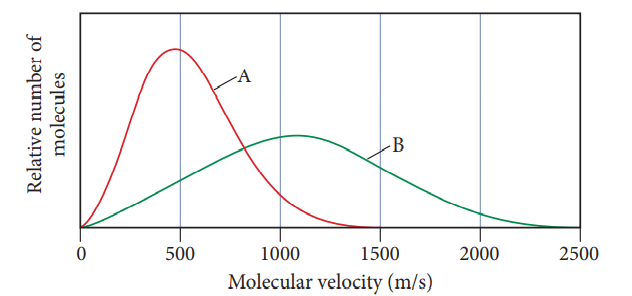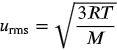1
188
views
59
Problem

# Problem 59

## Chapter 5: GasesTextbook ExpertVerified Tutor
26 Oct 2021

#### Given information

The graph showing the distribution of the molecular velocity of A and B gases is -:#### Step-by-step explanation

Step 1.

Formula of root mean square velocity.As per the given formula, the root mean square velocity of gas molecules is inversely proportional to the square root of the molar mass of the gas. Since the root mean square speed of gas A is less than gas B. Therefore, gas A has a higher molar mass than B.

The rate of effusion of the gas is inversely proportional to the square root of the molar mass of the gas. Therefore, the rate of effusion of B is higher than A as it has a lower molar mass.